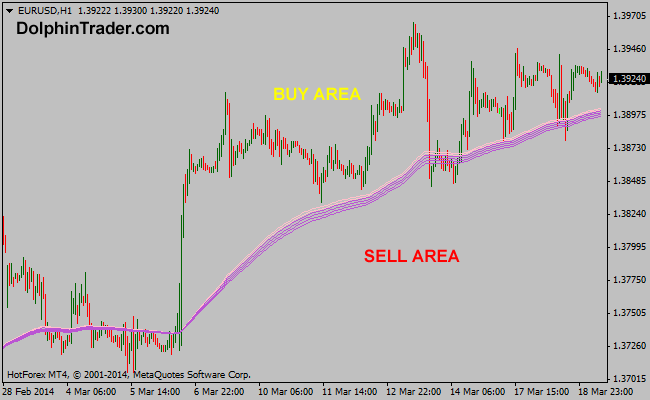# 118 EMA – 140 EMA Guppy Multi Moving Average Metatrader 4 Indicator

This is the third GMMA funnel indicator in a series of 11 indicators entirely based on the Guppy multi moving average. The RainbowMMA_03 consists of the following moving averages: 140 period exponential moving average, 135 period exponential moving average, 130 period exponential moving average, 125 period exponential moving average, 122 period exponential moving average and 118 period exponential moving average.

Buy: Wait for the price to rise above the RainbowMMA_03 GMMA
Sell: Wait for the price to drop below the RainbowMMA_03 GMMA

Indicator Preferences

Currency pairs: any
Time frames: any
Sessions: any